# AP Statistics : Random Variables

## Example Questions

1 3 Next →

Explanation:

### Example Question #1 : How To Do The Linear Transformation Of A Random Variable

Variablehas a Poisson distribution with a mean of. What is the variance of Variable?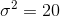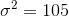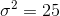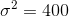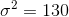Explanation:

Becausehas a Poisson distribution we know that: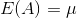and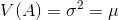.

Therefore, since we are given the mean of 25, we can find its variance to also be 25.

1 3 Next →

### All AP Statistics Resources